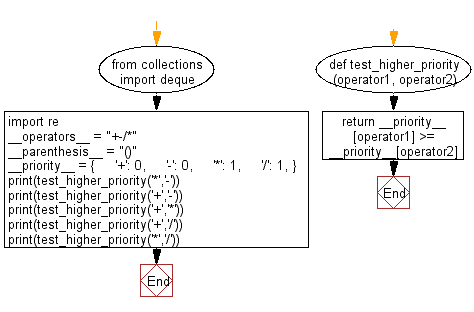﻿ Python: Check the priority of the four operators - w3resource

# Python: Check the priority of the four operators

## Python Basic - 1: Exercise-15 with Solution

Write a Python program to check the priority of the four operators (+, -, *, /).

Sample Solution:

Python Code:

``````from collections import deque
import re

__operators__ = "+-/*"
__parenthesis__ = "()"
__priority__ = {
'+': 0,
'-': 0,
'*': 1,
'/': 1,
}

def test_higher_priority(operator1, operator2):
return __priority__[operator1] >= __priority__[operator2]

print(test_higher_priority('*','-'))
print(test_higher_priority('+','-'))
print(test_higher_priority('+','*'))
print(test_higher_priority('+','/'))
print(test_higher_priority('*','/'))
``````

Sample Output:

```True
True
False
False
True
```

Flowchart:Python Code Editor :

Have another way to solve this solution? Contribute your code (and comments) through Disqus.

What is the difficulty level of this exercise?

Test your Programming skills with w3resource's quiz.

﻿